# Odd and even permutations

A standard part of the theory of permutations is the classification of permutations into “odd” and “even” types. In this post I will develop the theory of odd and even permutations, focusing on adjacent permutations.

Letbe an finite ordered set. A permutation ofis a rearrangement of; formally, it is a bijection. For simplicity, we will label the elements ofas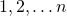, and we will represent a permutation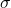by the list. Thus ifhas five elements, then the permutation that reversescan be written as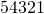.

The set of permutations ofnaturally forms a group. That is, given two permutations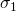and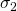, we can form their product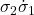(or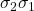for short), which is defined by performingfirst and then, following the convention for composition of functions. For example, the product of the permutations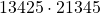is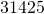.

Here are some standard permutations. The identity permutation is the permutation that does nothing. A transposition is a permutation that swaps two elements. If a transposition swaps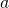and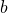, we will denote it as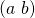. An adjacent transposition is a transposition that swaps two adjacent elements (so it is denoted).

An important property of a permutation is its inversion number. An inversion of a permutationis a pair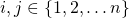where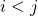such that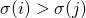. In other words, it is a pair of elements whose relative positions have been switched by the permutation. The inversion number ofis the size of its set of inversions. Thus the inversion number is at most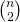(this occurs when the permutation reverses). A permutation has inversion number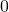if and only if it is the identity permutation. Also, a permutation has inversion numberif and only if it is an adjacent transposition.

A permutation is called odd if its inversion number is odd, and even if its inversion number is even. We would like to show that the product of odd and even permutations behaves like addition of odd and even numbers. To show this, we will use the following lemma:

Every permutation is a product of adjacent transpositions.

This can be proved by using insertion sort, an algorithm which effectively writes any permutations as a product of adjacent transpositions.

Here is how it works. Consider, for example, the permutationgiven by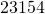. Let us try to sort this list. Insertion sort first movesto the front by repeated swaps, first swapping the second and third position and then the first and second positions. Then we get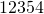. Then insertion sort would swap the fourth and fifth positions, and then finish with. So, if we do these transpositions in reverse, we get our original permutation. That is,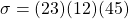. We can see that using this process, insertion sort can write any permutation as a product of adjacent transpositions.

We need one more lemma:

Ifis a permutation and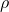an adjacent transposition, thenand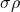have opposite parity. That is, one is odd and one is even.

Here is why. After performing, if we swap two adjacent elementsand, then the only change to the set of inversions is, sinceandhaven’t moved relative to any other element. So, the only possible change is thatwas an inversion and no longer is, or it wasn’t an inversion and now is. So the inversion number has either been increased or decreased by one – so the parity has been switched.

So, if a permutationis written as a product of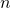adjacent transpositions, then its parity is equal to the parity of. This establishes that the product of odd and even permutations behaves like addition of odd and even numbers (formally, what we are saying is that “parity” defines a homomorphism from the permutation group to).

Another interesting fact is that every transposition is odd. We can see this (without needing to count inversions) by noticing that we can obtain any transposition(with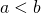) by repeatedly swapping adjacent elements till the element in placeis moved to place, and then repeatedly swapping the other direction till the element originally in placeis moved to place. If we write this out we see that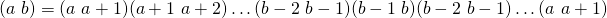, which has an odd number of terms.

Thus every permutation can be written only either as a product of an odd number or an even number of transpositions. Since the identity permutation is clearly even, we have the following remarkable fact: given any list, it is impossible, after performing an odd number of swaps, to obtain the original list.

Acknowledgements: Thanks to Anton Cao for suggestions. Thanks to Jessica Meng for reminding me the correct convention for composition order.